# Review: Mathematical socks

Can you wear them and be taken mathematically seriously?This Christmas, I received mathematical socks. A great gift, you might think. But is it good maths or fake maths? Can you wear them and be taken mathematically seriously? Thus I have undertaken this important review.

## Unboxing

The socks come beautifully packaged and folded, tied together with a fancy red label, which gives a nifty standing suggestion.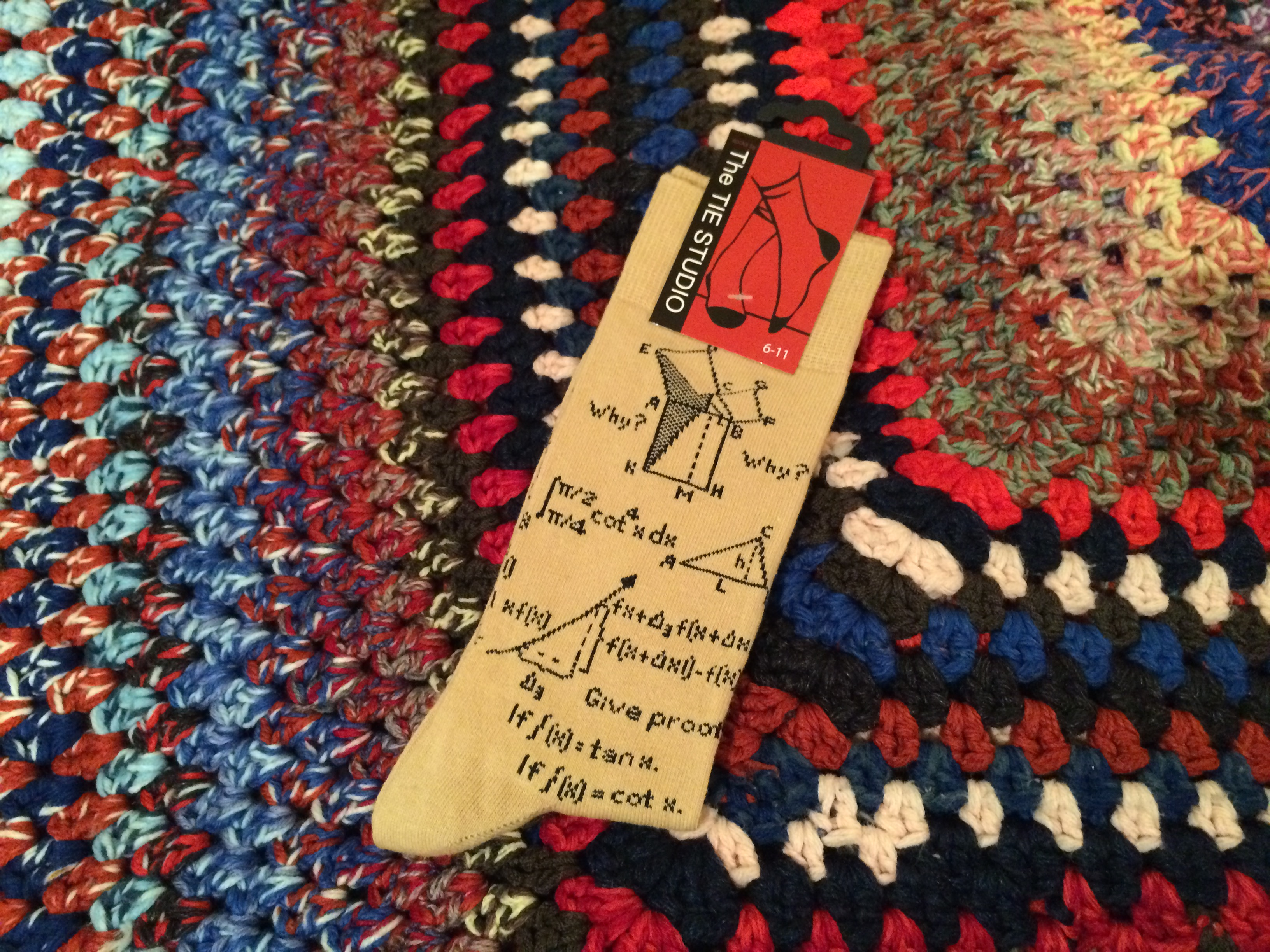Beautifully packed mathematical socks

## Mathematical content

There are five distinct mathematical items on the socks. I have graded them individually. The younger reader may wish to refer to this helpful guide to converting to new grades.

#### 1. Proof of Pythagoras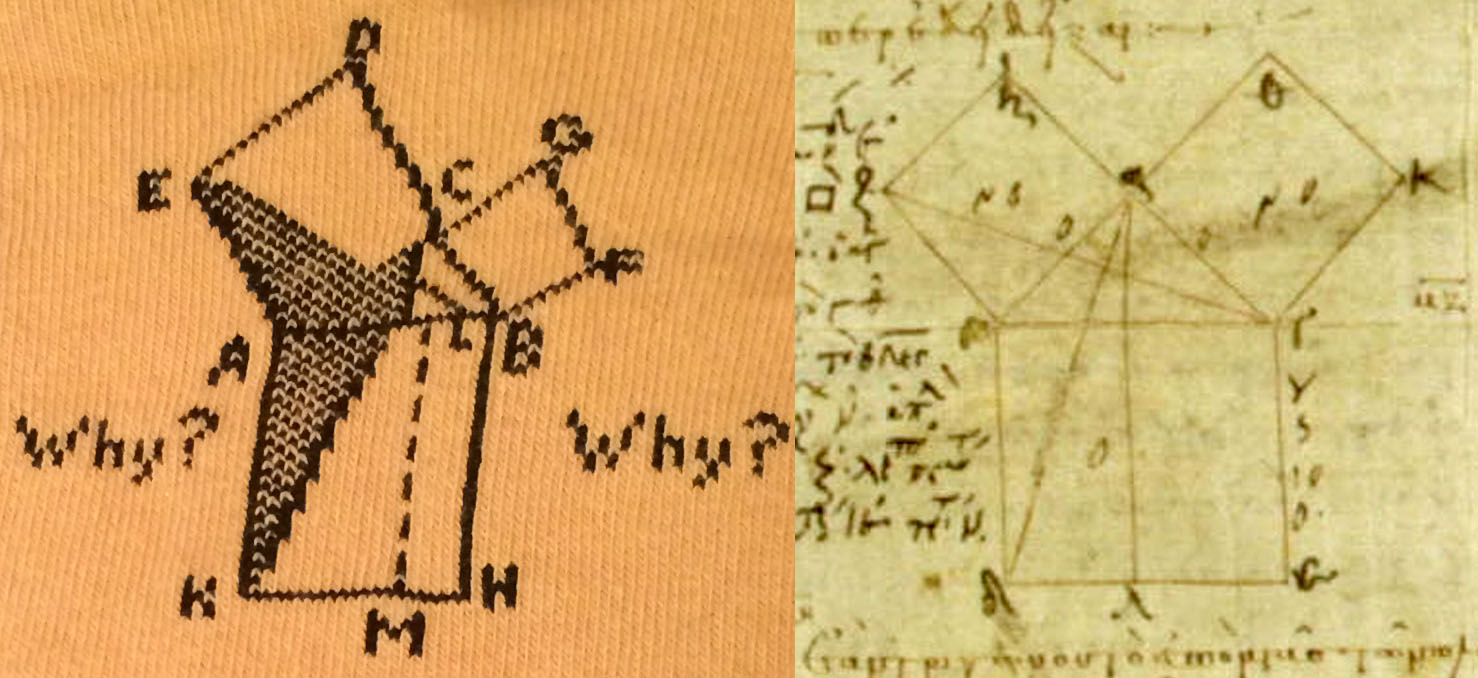Pythagoras’ Theorem proved on a sock (left) and in the Elements (right)

Not just any proof of Pythagoras, this is the proof from Euclid’s Elements!

Except they’ve only gone and ballsed it up. The dotted line from M to L should continue up to C instead of going off to the left. And the diagonal from E heading towards B really ought to get there instead of shifting up and somehow only reaching L?!

Why? Why?Maths grade: B. Close but no cigar.

#### 2. Definite integral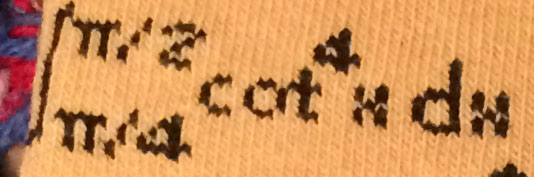Nice typography here: flat fractions in limits increases legibility. Surprisingly difficult integral, actually, but it submits to a trig identity and a couple of substitutions.
$$\int_{\pi/4}^{\pi/2} \cot^4 x \, \mathrm{d}x = \frac{\pi}{4} – \frac{2}{3}.$$Maths grade: A. Nice problem.

#### 3. Height of triangleConsider a triangle $ABC$. Let it have height $h$ and base $L$. Very good. Possibly related to the Pythagoras bit above.

#### 4. Derivation of differentation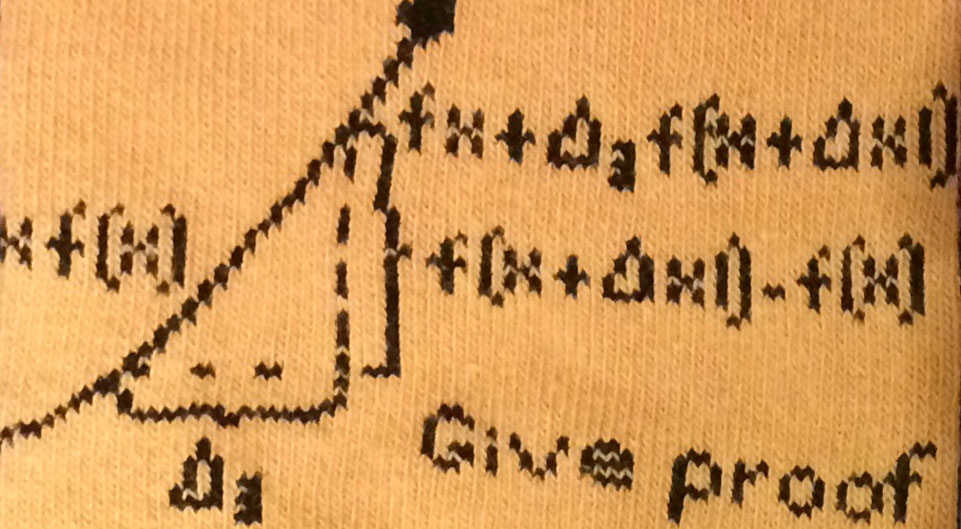Take a function $f(x)$ and then go a distance $\Delta_3$ (?!) to the right. Then the change in $f(x)$ is
$$f(x + \Delta x|) – f(x),$$which is almost right. Unfortunately it is then surrounded by
$$xf(x) \qquad fx + \Delta_3 f(x+\Delta x|)$$
But at least they have the humour to follow it with “Give proof”. LOL.
Maths grade: C. Some understanding shown.

#### 5. Indefinite integrals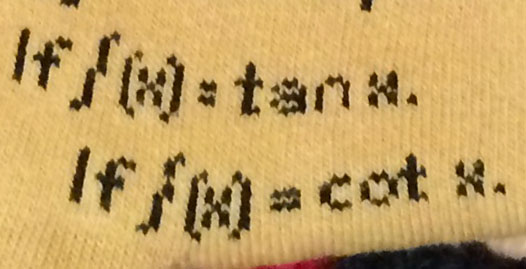$$\mathrm{If}\;\int(x) = \tan x, \qquad\mathrm{If}\; \int(x) = \cot x.$$You what mate?

## Fashion

Beige socks with triangles on them in black. A surefire hit, surely?

## Fit

Since the socks fit sizes 6–11 (??7–12, ??39–46), the concerned reader might think that the fit is not very good. But on my size-9 feet, I found the fit adequate, although I found they did go a surprisingly long way up my leg.

## UtilityBeautiful Sutton Park

I took the socks for a walk round beautiful Sutton Park, the seventh-largest urban park in Europe, on a cold wintery day.

My feet were only moderately cold and damp after two hours strolling around this beautiful mix of heathland, wetlands and marshes, seven lakes and extensive ancient woodlands, which is larger than Richmond Park. OK but probably best for the spring and summer.

## Overall review

Choice buffer between foot and shoe.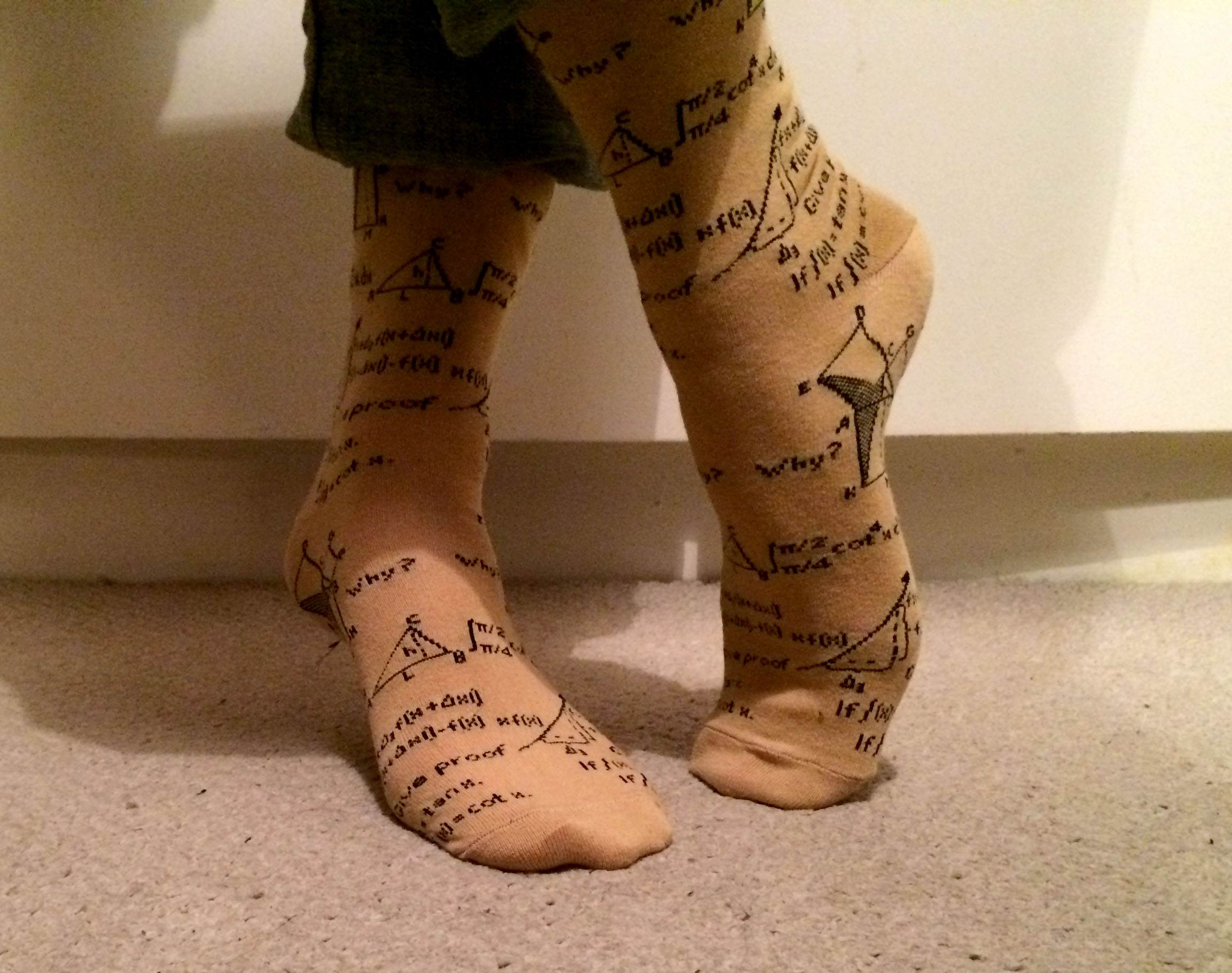NiftyAdam is a postdoctoral researcher at Imperial College London, where he investigates weird, non-Newtonian fluids. If he’s not talking about the maths of chocolate fountains he is probably thinking about fonts, helping Professor Dirichlet answer your personal problems, and/or listening to BBC Radio 2.